ENGR338 Lab 2022 Fall
Lab 4
MOSFETs and IV Curves

MOSFETs and IV Curves

Introduction: This lab uses Electric VLSI and LTSpice to simulate pmos and nmos gates.

Materials: LTSpice, Electric VLSI

Methods: First, an NMOS was placed and the spice-model was set to NMOS as per the simulation file. This was repeated for the PMOS as well before layouts of each were made.

The layout was made using and nMos node and two nAct nodes which functioned as the drain/sink for the node. A metal1 to poly pin was placed allowing a metal 1 arc to be used to connect to the gate. For the NMOS node, a pWell was used to ground the gate to the body of the chip. The width of every component(except the metal1-poly pin) was set to 10 before the nodes were connected as seen below.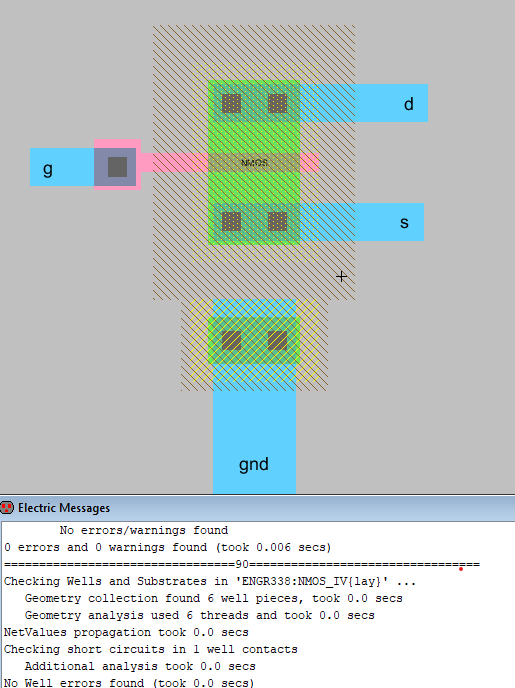Figure 1. NMOS layout completed with 0 errors.

The same process was completed for the PMOS circuit using an nWell for ground and pAct/pMos accordingly. This can be seen below in Figure 2.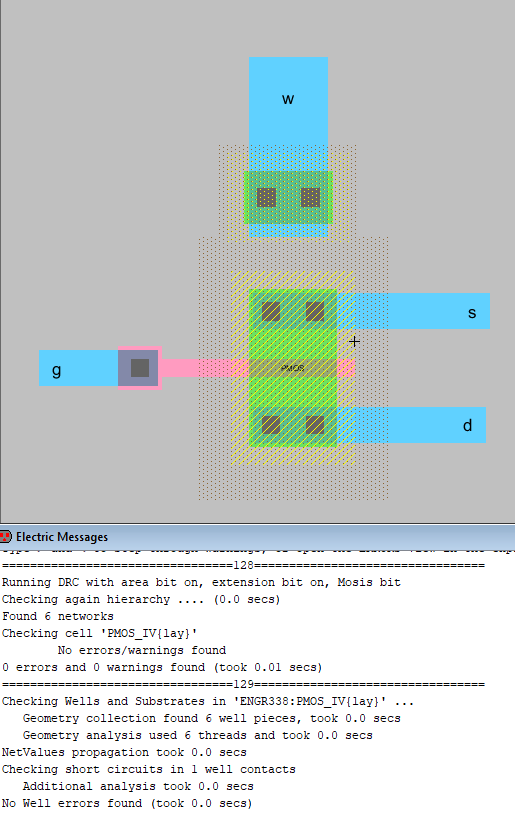Figure 2. NMOS layout completed with 0 errors.

Both of these layouts passed both DRC and NCC checks as seen in the screenshots and were simulated in LTSpice which can be seen in the Results section(Figure 4 and 5).

The schematics were then completed similar to the layouts so that they had connections and the whole thing passed NCC. The connections were straight forward and can be seen below in Figure 3 and Figure 4.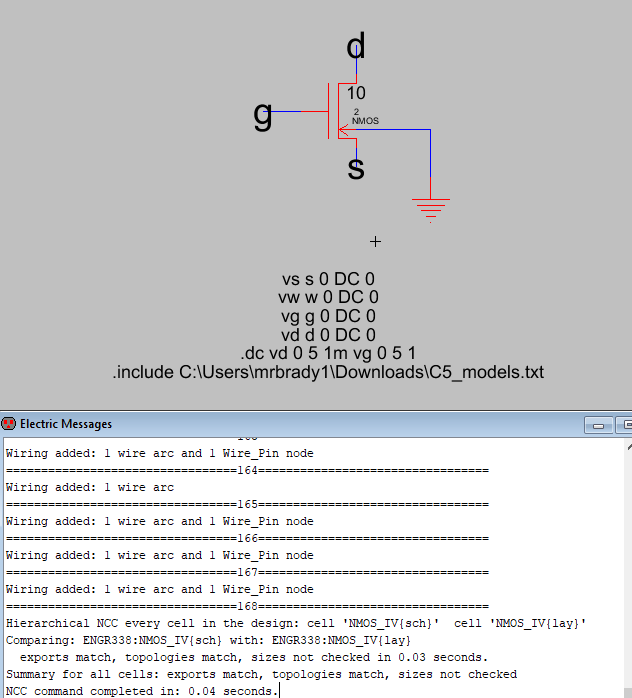Figure 3. Nmos schematic connections and NCC results.

This was fairly straightforward and simulation code was written to simulate the file. These results were the same as the original simulation and can be seen below.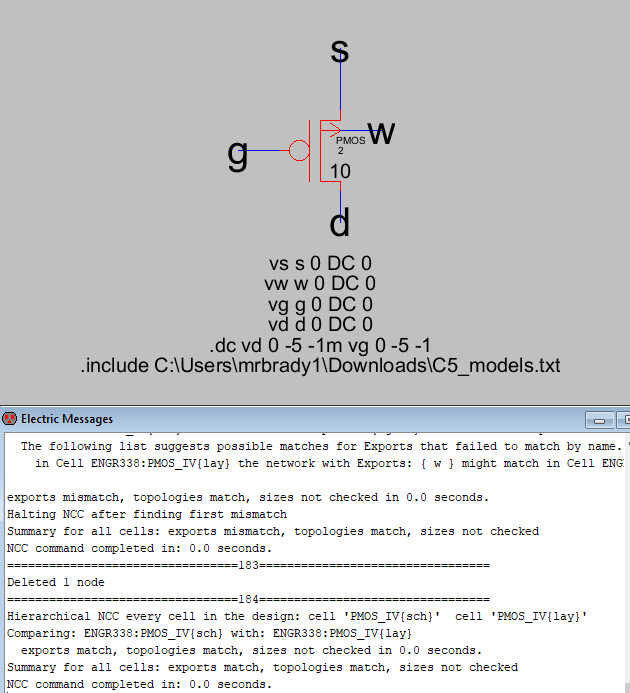Figure 3. Pmos schematic connections and NCC results.

Results:

Both circuits were simulated and got the following results.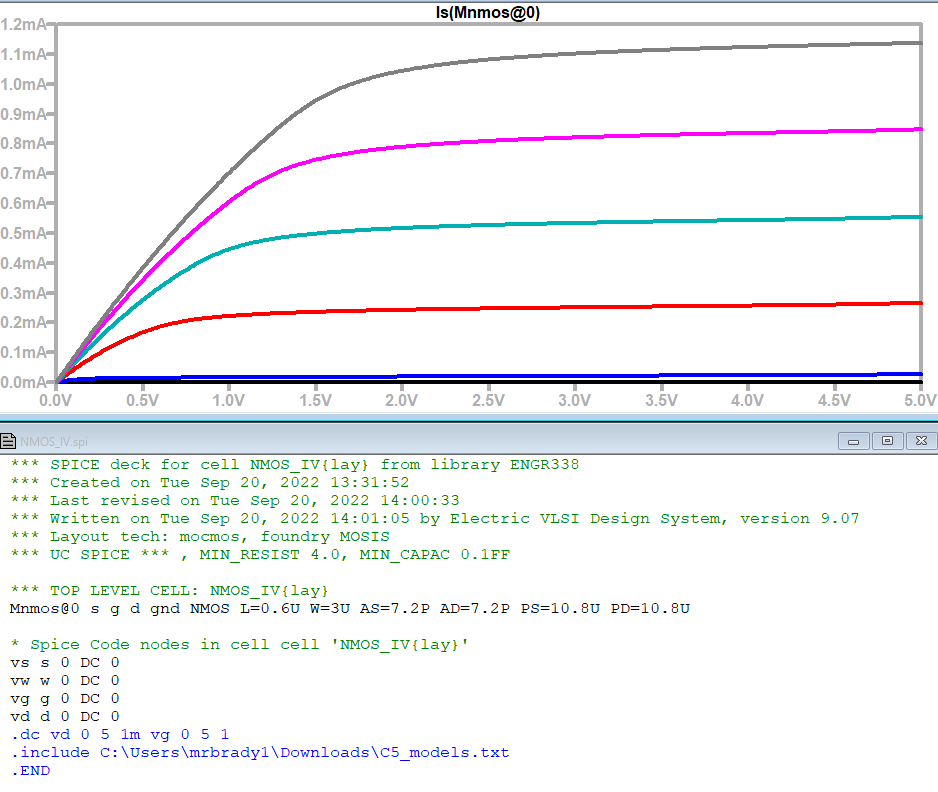Figure 4. Simulation results for NMOS transistor as Vd and Vg were changed from 0 to 5V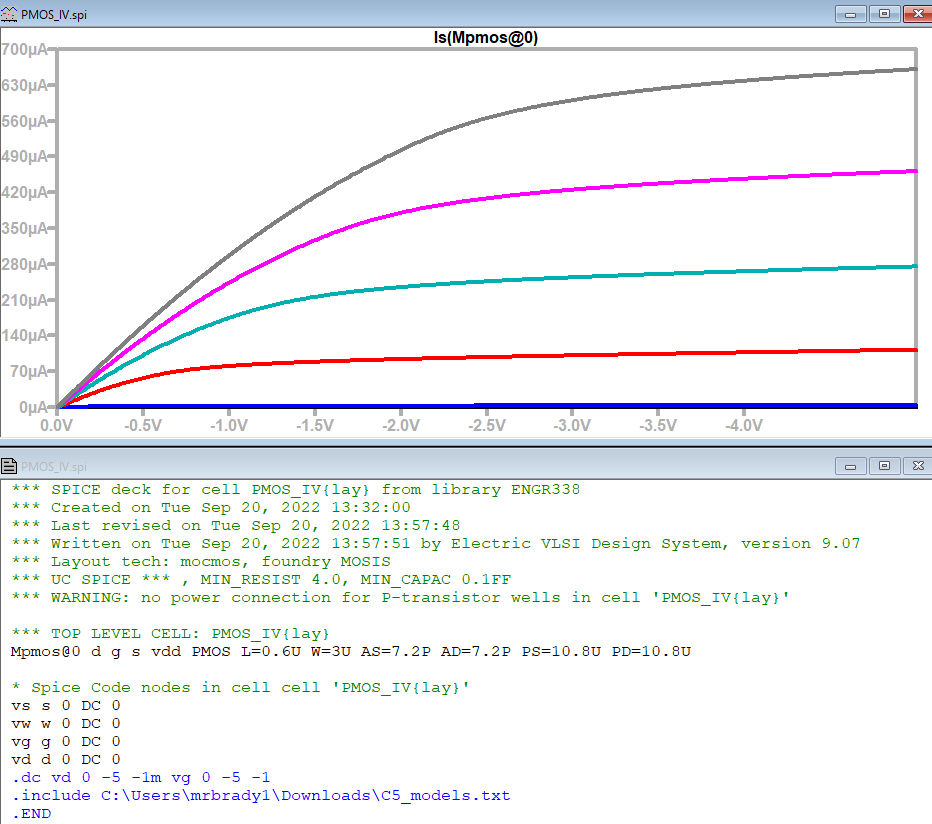Figure 4. Simulation results for PMOS transistor as Vd and Vg were changed from 0 to 5V

Discussion:
The simulation results were a little weird. I flipped the voltages to negative for some reason in my PMOS circuit which resulted in me getting nearly identical graphs between the two. I'm not sure what my logic behind this was but the true graphs should be mirror images of each other.